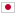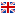Contest Duration: - (local time) (300 minutes)
D - Parentheses/Time Limit: 2 sec / Memory Limit: 512 MB

### Problem Statement

Dave loves strings consisting only of '(' and ')'. Especially, he is interested in balanced strings. Any balanced strings can be constructed using the following rules:

• A string "()" is balanced.
• Concatenation of two balanced strings are balanced.
• If $T$ is a balanced string, concatenation of '(', $T$, and ')' in this order is balanced. For example, "()()" and "(()())" are balanced strings. ")(" and ")()(()" are not balanced strings.

Dave has a string consisting only of '(' and ')'. It satisfies the followings:

• You can make it balanced by swapping adjacent characters exactly $A$ times.
• For any non-negative integer $B~(B \lt A)$, you cannot make it balanced by $B$ swaps of adjacent characters.
• It is the shortest of all strings satisfying the above conditions.

Your task is to compute Dave's string. If there are multiple candidates, output the minimum in lexicographic order. As is the case with ASCII, '(' is less than ')'.

### Input

The input consists of a single test case, which contains an integer $A \,(1 \le A \le 10^9)$.

### Output

Output Dave's string in one line. If there are multiple candidates, output the minimum in lexicographic order.

### Sample Input 1

1

### Output for the Sample Input 1

)(

There are infinitely many strings which can be balanced by only one swap. Dave's string is the shortest of them.

### Sample Input 2

4

### Output for the Sample Input 2

)())((

String "))(()(" can be balanced by $4$ swaps, but the output should be ")())((" because it is the minimum in lexicographic order.